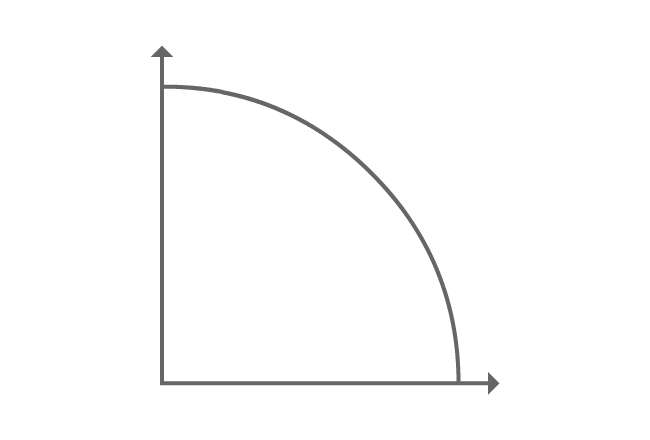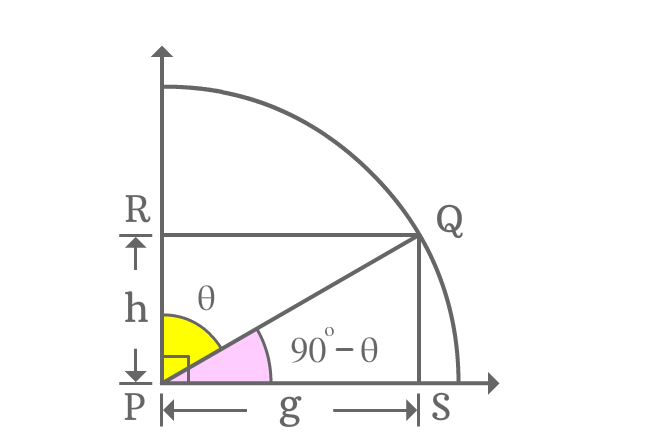# Cofunction identity of cot function

## Formula

Sexagesimal System
$\cot{(90^\circ-\theta)} \,=\, \tan{\theta}$
$\cot{\Big(\dfrac{\pi}{2}-x\Big)} \,=\, \tan{x}$

Theta ($\theta$) is taken as angle in degrees and $x$ is taken as an angle in radians in this law.

Cot function has an allied angle and it belongs to first quadrant. Hence, this formula is recognized as first quadrant’s allied angle identity of cot function. The angles of both reciprocal functions are complementary angles. So, this trigonometric rule is generally called as cofunction identity of cot function.

### ProofA line segment is rotated to an angle in first quadrant and is represented by $\overline{PQ}$.

In first quadrant, lines are drawn perpendicular to the horizontal and vertical rays from point $Q$ and they intersect the both horizontal and vertical rays at points $S$ and $R$ respectively. Geometrically, it formed two right angled triangles, known as $\Delta QPR$ and $\Delta QPS$.

Take $\angle QPR = \theta$, then $\angle QPS = 90^\circ-\theta$.

If $PS = g$, then $RQ = g$ and if $RP = h$, then $QS = h$ because of parallelism and equality of the line segments.

#### Express cot of allied angle$90^\circ-\theta$ is an allied angle of the $\Delta QPS$. Now, express cot of allied angle of first quadrant as a ratio of lengths of the respective sides.

$\cot{(90^\circ-\theta)} = \dfrac{PS}{QS}$

The lengths of both adjacent and opposite sides are known, and they are $g$ and $h$ respectively.

$\implies \cot{(90^\circ-\theta)} = \dfrac{g}{h}$

#### Find equivalent value of the fractionCot of allied angle of first quadrant is written as a fraction of the lengths of the respective sides. So, try to write the fraction as its equivalent trigonometric function. Possibly, it can be done by considering $\Delta QPR$.

$\dfrac{g}{h} = \dfrac{PS}{QS}$

The angle of the triangle $\Delta QPR$ is theta. The ratio of lengths of $\overline{PS}$ to $\overline{QS}$ is tan of angle theta.

$\implies \dfrac{g}{h} = \tan{\theta}$

#### Cofunction identity of cot function

The above two steps proved geometrically that

$\cot{(90^\circ-\theta)}$ $=$ $\dfrac{g}{h}$ $=$ $\tan{\theta}$

$\therefore \,\,\,\,\,\, \cot{(90^\circ-\theta)} \,=\, \tan{\theta}$

It is proved that cot of allied angle of first quadrant is equal to tan of angle. So, this rule of trigonometry is known as first quadrant’s allied angle identity of cot function.

The angles of both cot and tan functions are complementary. Therefore, this trigonometric identity is also known as cofunction identity of cot function.

Latest Math Topics
Jun 26, 2023
Jun 23, 2023

###### Math Questions

The math problems with solutions to learn how to solve a problem.

Learn solutions

Practice now

###### Math Videos

The math videos tutorials with visual graphics to learn every concept.

Watch now

###### Subscribe us

Get the latest math updates from the Math Doubts by subscribing us.